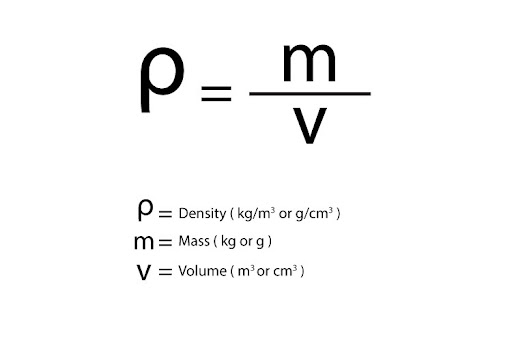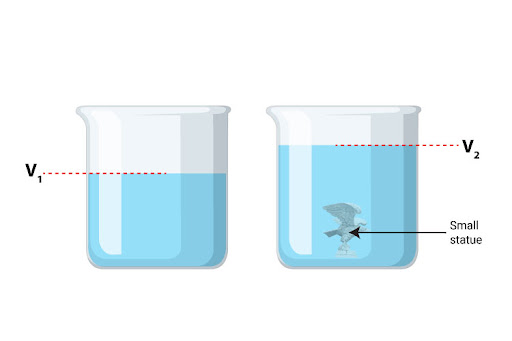# Suppose a student wants calculate the density of the two different objects, a metal cube and a small statue. Describe the methods the student could use to measure the density of the two objects.

Let us first define density as the amount of mass contained per unit volume of an object. It is technically a ratio, and is calculated using the formula:So it is clear how we only need two components to calculate density: mass and volume. For a metal cube, we measure its mass by simply placing it on a mass balance. As for its volume, we’ll take advantage of the fact that a cube is regularly shaped - so just by measuring one side’s length with a ruler, all we then have to do is use the formula for the volume of a cube: V = l3. After that, it is simply a matter of dividing mass by volume!

The statue’s mass can be measured easily with a mass balance, the story is a little different for its volume, considering that it’s no longer regularly shaped - there’s no strict formula we can use! We do, however, have a different technique called the displacement method (or more interestingly, the Eureka method!) The steps are as follows:

1. First, fill up a measuring cylinder approximately halfway and measure this initial volume (V1)
2. Then, place the small statue inside, and measure the new volume (V2)
3. Note that this new volume is simply the initial water volume combined with the statue’s own volume - therefore, volume of the statue is simply: Vs = V2 - V1After that, just divide mass by volume, and we’ll have the statue’s density!